# New SAT Math - Calculator : Solving Word Problems with Multiple Unit Conversions

## Example Questions

### Example Question #1 : Solving Word Problems With Multiple Unit Conversions

How many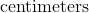are in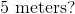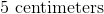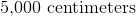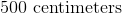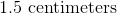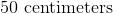Explanation:

To solve this problem we can make proportions.

We know that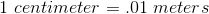and we can useas our unknown.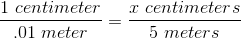Next, we want to cross multiply and divide to isolate the  on one side.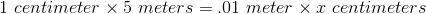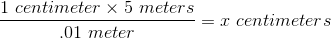The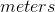will cancel and we are left with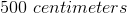### Example Question #2 : Solving Word Problems With Multiple Unit Conversions

How many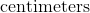are in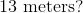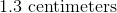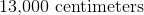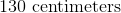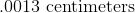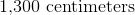Explanation:

To solve this problem we can make proportions.

We know thatand we can useas our unknown.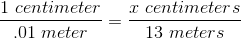Next, we want to cross multiply and divide to isolate the  on one side.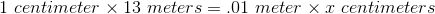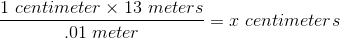Thewill cancel and we are left with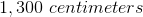### All New SAT Math - Calculator Resources